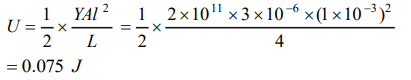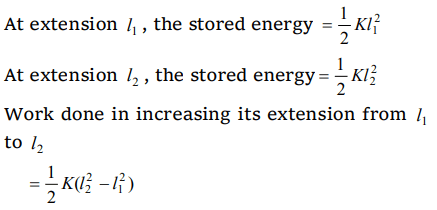## Mechanical Properties of Solids Questions and Answers Part-18

1. The ratio of Young's modulus of the material of two wires is 2 : 3. If the same stress is applied on both, then the ratio of elastic energy per unit volume will be
a) 3 : 2
b) 2 : 3
c) 3 : 4
d) 4 : 3

Explanation:2. The length of a rod is 20 cm and area of crosssection $2c m^{2}$ . The Young's modulus of the material of wire is $1.4\times 10^{11}N\diagup m^{2}$  . If the rod is compressed by 5 kg-wt along its length, then increase in the energy of the rod in joules will be
a) $8.57\times 10^{-6}$
b) $22.5\times 10^{-4}$
c) $9.8\times 10^{-5}$
d) $45.0\times 10^{-5}$

Explanation:3. If a spring extends by x on loading, then the energy stored by the spring is (if T is tension in the spring and k is spring constant)
a) $\frac{T^{2}}{2x}$
b) $\frac{T^{2}}{2k}$
c) $\frac{2x}{T^{2}}$
d) $\frac{2T^{2}}{k}$

Explanation:4.On stretching a wire, the elastic energy stored per unit volume is
a) $Fl\diagup 2AL$
b) $FA\diagup 2L$
c) $FL\diagup 2A$
d) $FL\diagup 2$

Explanation:5. When a force is applied on a wire of uniform cross-sectional area $3\times 10^{-6}m^{2}$  and length 4m, the increase in length is 1 mm. Energy stored in it will be $\left(Y=2\times 10^{11}N\diagup m^{2}\right)$
a) 6250 J
b) 0.177 J
c) 0.075 J
d) 0.150 J

Explanation:6. K is the force constant of a spring. The work done in increasing its extension from $l_{1}$ to$l_{2}$ will be
a) $K\left(l_{2}-l_{1}\right)$
b) $\frac{K}{2}\left(l_{2}+l_{1}\right)$
c) $K\left(l_2^2-l_1^2\right)$
d) $\frac{K}{2}\left(l_2^2-l_1^2\right)$

Explanation:7. When a 4 kg mass is hung vertically on a light spring that obeys Hooke's law, the spring stretches by 2 cms. The work required to be done by an external agent in stretching this spring by 5 cms will be $\left(g= 9.8 m \diagup sec^{2}\right)$
a) 4.900 joule
b) 2.450 joule
c) 0.495 joule
d) 0.245 joule

Explanation:8. A wire of length L and cross-sectional area A is made of a material of Young's modulus Y. It is stretched by an amount x. The work done is
a) $\frac{YxA}{2L}$
b) $\frac{Yx^{2}A}{L}$
c) $\frac{Yx^{2}A}{2L}$
d) $\frac{2Yx^{2}A}{L}$

Explanation: $\frac{Yx^{2}A}{2L}$

9. The elastic energy stored in a wire of Young's modulus Y is
a) $Y\times\frac{Strain^{2}}{Volume}$
b) $Stress\times Strain \times Volume$
c) $\frac{Strain^{2}\times Volume}{2Y}$
d) $\frac{1}{2} \times Y \times Stress\times Strain \times Volume$

Explanation: $\frac{Strain^{2}\times Volume}{2Y}$
10. A wire of length 50 cm and cross sectional area of 1 sq. mm is extended by 1 mm. The required work will be $\left(Y=2\times10^{10}N m^{-2}\right)$
a) $6\times10^{-2}J$
b) $4\times10^{-2}J$
c) $2\times10^{-2}J$
d) $1\times10^{-2}J$